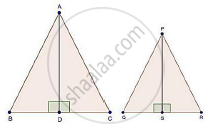Share

# The Areas of Two Similar Triangles Are 81 Cm2 and 49 Cm2 Respectively. Find the Ratio of Their Corresponding Heights. What is the Ratio of Their Corresponding Medians? - CBSE Class 10 - Mathematics

#### Question

The areas of two similar triangles are 81 cm2 and 49 cm2 respectively. Find the ratio of their corresponding heights. What is the ratio of their corresponding medians?#### Solution

We have,

ΔABC ~ ΔPQR

Area (ΔABC) = 81 cm2,

Area (ΔPQR) = 49 cm2

And AD and PS are the altitudes

By area of similar triangle theorem

("Area"(triangleABC))/("Area"(trianglePQR))="AB"^2/"PQ"^2

rArr81/49="AB"^2/"PQ"^2

rArr9/7="AB"/"PQ"                          ….(i) [Taking square root]

𝐼𝑛 ΔABD and ΔPQS

∠B = ∠Q                              [ΔABC ~ ΔPQR]

∠ ADB = ∠PSQ                     [Each 90°]

Then, ΔABD ~ ΔPQS            [By AA similarity]

therefore"AB"/"PQ"="AD"/"PS"               …(ii) [Corresponding parts of similar Δ are proportional]

Compare (1) and (2)

"AD"/"PS"=9/7

∴ Ratio of altitudes = 9/7

Since, the ratio of the area of two similar triangles is equal to the ratio of the squares of the squares of their corresponding altitudes and is also equal to the squares of their corresponding medians.

Hence, ratio of altitudes = Ratio of medians = 9 : 7

Is there an error in this question or solution?

#### Video TutorialsVIEW ALL 

Solution The Areas of Two Similar Triangles Are 81 Cm2 and 49 Cm2 Respectively. Find the Ratio of Their Corresponding Heights. What is the Ratio of Their Corresponding Medians? Concept: Areas of Similar Triangles.
S